## Mechanical Comprehension Practice Test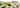# Mechanical Comprehension Test

Updated 4 July 2021

## Mechanical Comprehension Practice Test

Mechanical reasoning testsmeasure your knowledge of mechanical and physical concepts. They do not measure your underlying mechanical aptitude in the same way that abstract reasoning questions measure your underlying intellectual ability.

For example, you could sit an abstract reasoning test without having seen one before and still get a reasonable score. The same is not true of mechanical reasoning, where your score will depend significantly on your knowledge of:

• Levers
• Pulleys
• Gears
• Springs
• Simple Electrical Circuits
• Tools
• Arithmetic

You may have come across many of these topics in elementary science and the questions will seem fairly straightforward. If elementary science classes seem like a long time ago, then you'll need to refresh your memory.

This article also covers fault diagnosis questions which are used to select personnel for technical roles where they need to be able to find and repair faults in electronic control systems.

The questions in fault diagnosis tests appear rather abstract and require pure logic to solve. This is because when a fault develops in an electronically controlled system, there is often no physical clue as to the cause. In the absence of such a clue, for example, an obviously burned-out component, the only way to diagnose the problem is by a process of logical elimination.

## Practice Mechanical Test 1

To help you get an idea of what a mechanical reasoning test involves, here is a practice test to get you started.

Credit: Psychometric Success

## What to Expect on a Mechanical Aptitude Test

Mechanical reasoning questions vary widely in difficulty from test to test.

If you are taking a mechanical reasoning test as part of the selection process for the emergency services or the military, then the questions will tend to concentrate on principles rather than on making calculations. For example, you may be shown three diagrams of a lever and asked which one is the most efficient.

If, however, you are taking a test for a craft or technical job, you may be expected to calculate the actual force required to move a particular lever. In this case, knowing the principle is not enough; you need to know the formula. You may also be asked some questions about tools and how they are used.

If you are taking a test as part of the selection for a craft or apprenticeship job, you should also expect some basic arithmetic questions. These questions approximate the type of reasoning and maths that are needed to estimate materials costs, etc.

One final point: many of the questions in mechanical reasoning tests are industry-specific. For example, tests used by the fire service will tend to frame questions in terms of firefighting, whereas tests used to select for an aircraft maintenance job would tend to frame the questions in aviation industry terms.

It doesn’t matter if the questions you practice on aren’t specific to the industry you are applying for. It is the substance of the question that is important – the scenario is incidental.

## Mechanical Reasoning Question Types

Mechanical reasoning tests are designed to assess your knowledge of physical and mechanical principles. Questions are in the form of a question and a diagram, and you will need to determine which mechanical principle is being illustrated.

No specialist knowledge is required to answer these questions, only an understanding of basic mechanical and physical principles.

Mechanical reasoning tests are used to select for a wide range of jobs including the military, police force and fire service, as well as various engineering occupations. These tests are usually of 20–30 minutes duration and contain 20–30 questions that concentrate on the following:

• Levers
• Pulleys
• Gears
• Springs
• Gravity
• Electricity
• Magnetism
• Linear Force
• Centrifugal Force
• Centripetal Force
• Compression
• Expansion

### Levers

A lever consists of a bar that pivots at a fixed point known as the fulcrum. In the example shown, the fulcrum is at the centre of the lever. This lever provides no mechanical advantage and the force needed to lift the weight is equal to the weight itself.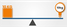Mechanical Comprehension Test

However, if you want to lift a weight that is heavier than the force applied you can move the fulcrum closer to the weight to be lifted.

This affects the force required in the following way:

w x d1 = f x d2

Where:

• w = weight
• d1 = distance from fulcrum to weight
• f = force needed
• d2 = distance from the fulcrum to the point where force is applied

In this example, the fulcrum has been moved towards the weight, so that the weight is one metre from the fulcrum. This means that the force can now be applied two metres from the fulcrum.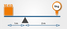Mechanical Comprehension Test

If you needed to calculate the force needed to lift the weight then you can rearrange the formula:

w x d1 = f x d2 can be rearranged to f = (w x d1)/d2

f = (10 x 1)/2 (10/2 is the same as 5/1, the force required is 5 kg)

How much force is required to lift the weight?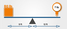Mechanical Comprehension Test

A) 40 lbs
B) 50 lbs
C) 60 lbs
D) 70 lbs

Answer: C – 60 lbs is needed to lift the weight. It can be calculated like this:

f = (w x d1)/d2
f = (80 x 9)/12
f = (720)/12
f = 60 lbs

In practice, levers are used to reduce the force needed to move an object – in other words, to make the task easier. However, in mechanical aptitude questions, you may see questions where the fulcrum has been placed closer to the force than the weight. This will mean that a force greater than the weight will be required to lift it.

You may see more complex questions involving levers. For example, there may be more than one weight. In this case, you need to work out the force required to lift each weight independently and then add them together to get the total force required.

### Pulleys

The pulleys used in this type of question consist of a grooved wheel and a block that holds it. A rope runs in the groove around the wheel and one end will be attached to either:

• A weight
• A fixed object like the ceiling
• Another pulley

For these questions, you can ignore the effect of friction.

Which weight requires the least force to move?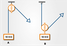Mechanical Comprehension Test

A) A
B) B
C) Both require the same force

Answer: B – Weight B requires a force equal to 5 kg, whereas A requires a force equal to 10 kg.

Single pulley questions are relatively straightforward. If the pulley is fixed then the force required is equal to the weight. If the pulley moves with the weight then the force is equal to half of the weight.

Another way of thinking about this is to divide the weight by the number of sections of rope supporting it, to obtain the force needed to lift it.

In A, there is only one section of rope supporting the weight, so 10/1 = 10 kg required to lift the weight.

In B, there are two sections of rope supporting the weight, so 10/2 = 5 kg required to lift it.

There are two possible ways that two pulleys can be used. Either one pulley can be attached to the weight or neither of them can be.

Which weight requires the least force to move?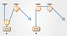Mechanical Comprehension Test

A) A
B) B
C) Both require the same force

Answer: A – Weight A requires a force equal to 5 kg, whereas weight B requires a force equal to 10 kg. Remember to divide the weight by the number of sections of rope supporting it, to get the force needed to lift the weight.

How much force is required to move the weight?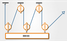Mechanical Comprehension Test

A) 100 kg
B) 150 kg
C) 50 kg
D) 60 kg

Answer: C – The weight is 300 kg and there are six sections of rope supporting it. Divide 300 by 6 to get 50 kg. In all cases, just divide the weight by the number of sections of rope supporting it to get the force needed to lift the weight.

### Gears

A gear is a toothed wheel or cylinder that meshes with another toothed component to transmit motion or to change speed or direction.

Gears are attached to a rotating shaft turned by an external force, which is not usually illustrated in these types of question. Two gears may be connected by touching each other directly or using a chain or belt. If gears are connected by a chain or belt then they move in the same direction.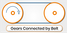Mechanical Comprehension Test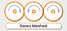Mechanical Comprehension Test

If the gears are touching (meshed) then adjacent gears move in opposite directions. In this example, the first and third gear will turn in the same direction. When there is an odd number of meshed gears, the last gear will always turn in the same direction as the first one.

Meshed gears with an equal number of teeth will turn at the same speed. If they have an unequal number of teeth, then the gear with the fewest teeth will turn faster. To work out how fast one is turning with respect to the other you need to count the teeth.

### Springs

A spring is a piece of wire or metal that can be extended or compressed by an external force, but which then returns to its original length when that force is no longer applied.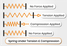Mechanical Comprehension Test

There are many different types of spring including:

• Spiral coil
• Leaf springs
• Torsion springs

Springs are used in many applications including clocks, vehicle suspensions, etc.

In the type of questions that you will be asked in mechanical aptitude tests, you can assume that springs behave in a linear way. That is, doubling the force applied will stretch or compress the spring twice as much.

If more than one spring is used then they can be arranged in one of two ways, either in series or in parallel.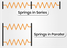Mechanical Comprehension Test

When springs are arranged in series, each spring is subjected to the force applied. When the springs are arranged in parallel, the force is divided equally between the springs.

Example Question

A force of 5 kg compresses the springs in series by 10 cm. What will be the total distance that the springs in parallel are compressed?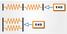Mechanical Comprehension Test

A) 10 cm
B) 2.5 cm
C) 5 cm
D) 7.5 cm

Answer: C – The total force will be divided equally between the two springs in parallel.

Since the force is divided in half, the distance moved will also be halved. The springs in series were compressed by 10 cm, therefore the springs in parallel will be compressed by 5 cm.

### Electricity

Questions on electricity usually take the form of simple circuit diagrams.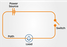Mechanical Comprehension Test

These diagrams are usually restricted to showing the power source, switches, loads (typically bulbs) and the path of the wiring. To answer these questions, you need a basic understanding of how electricity flows around a circuit.

How many switches need to be closed to light up one bulb?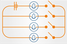Mechanical Comprehension Test

A) 1
B) 2
C) 3
D) 4

How many bulbs will light up when the switch is closed?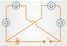Mechanical Comprehension Test

A) 1
B) 2
C) 3
D) 4

If bulb 1 is removed, how many bulbs will light up when the switch is closed?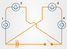Mechanical Comprehension Test

A) 1
B) 2
C) 3
D) 0

B – Two switches need to be closed to complete a circuit
D – All four bulbs will light up
B – Only bulbs 3 and 4 will light up

### Tools

If you are taking a test as part of the selection for a craft or apprenticeship job, then you may be asked some questions about tools and how they are used.

These questions are typically straightforward and if you have spent significant time fixing or making things, they won’t present any problems.

However, many people under 30 years of age don’t have much practical experience of fixing things or of watching someone else do so. If you feel that this applies to you, then you will need to make some effort to improve your knowledge of everyday tools. You can do this by getting hold of a catalogue for a tool hire company and simply reading through it.

### Other Things You May Need to Know

Some of the questions you come across may require knowledge of basic mathematics, such as:

These basic mathematical skills are an important aspect of mechanical ability. Making simple calculations based on the information provided and your knowledge of mechanical principles is part of these tests.

Some questions may also require you to know some simple formulas. For example:

The areas of shapes like rectangles, circles and triangles: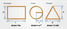Mechanical Comprehension Test

Some simple geometry like Pythagoras Theorem: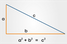Mechanical Comprehension Test

You will also need to be familiar with imperial units including inches, feet and yards. For example, you need to know that there are nine square feet in a square yard, something which surprises many people brought up using only metric units.

A good understanding of forces and energy are also essential, such as:

• Kinetic/potential energy
• Work and power
• Acceleration
• Gravity
• Pressure
• Friction

## Practice Mechanical Test 2

Credit: Psychometric Success

## Fault Diagnosis

Fault Diagnosis tests are used to select technical personnel who need to be able to find and repair faults in electronic and mechanical systems.

As modern equipment of all types becomes more dependent on electronic control systems (and, arguably, more complex), the ability to approach problems logically to find the cause of the fault is increasingly important.

This type of test is used extensively to select technical and maintenance personnel, as well as to select for artificer (technical) roles within the armed forces. For example, an aircraft technician.

This type of test usually forms part of a test battery in which verbal reasoning and numerical reasoningalso feature. No specialised knowledge is required to answer fault diagnosis questions (unlike mechanical reasoning).

Only when you are happy that you understand how these questions work should you attempt the practice tests. Remember that the object is to work systematically through the questions and avoid mistakes.

### Example Question

There are two components to this question:

1. A grid which describes the function of a series of switches
2. A flowchart showing: input – switches – output

Each of the switches acts to alter the input before the next switch in the series.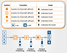Mechanical Comprehension Test

This grid shows four switches and their effect on four numbered inputs, which may be either ‘on’ or ‘off’.

The first switch (diamond symbol) inverts inputs one and two. That is, if the input is on, it is turned off and if it is off it is turned on. The other switches act in a similar way as detailed in the grid. If a switch is not working then it does not affect the input which passes through unchanged.

In this example flowchart, all of the inputs (numbered 1, 2, 3, 4) are on.

When these inputs pass through the first switch 1 & 3 are inverted (i.e. switched off).

These modified inputs (1 = off, 2 = on, 3 = off, 4 = on) then pass through the second switch.

The second switch inverts inputs 3 & 4 which gives (1 = off, 2 = on, 3 = on, 4 = off).

These modified inputs (1 = off, 2 = on, 3 = on, 4 = off) then pass through the third switch.

The third switch should invert inputs 2 & 4, giving (1 = off, 2 = off, 3 = on, 4 = on).

However, the third switch is not working so the output from switch two is not changed.

The answer to this question is, therefore, C, because switch type C is at fault.

## Fault Diagnosis Test 1

Credit: Psychometric Success

## Mechanical Reasoning Tests: Tips and Techniques

The most important thing is practice. Since mechanical aptitude tests are tests of your applied knowledge, it is really important to ensure that you have learned and practised the types of problems likely to be included.

A suggested list of topics to revise includes (but is not limited to):

• Levers
• Gears
• Pulleys
• Springs
• Screws
• Acceleration
• Gravity
• Clamps
• Shafts
• Pressure
• Friction
• Weights
• Volumes
• Conveyor belts
• Kinetic and potential energy
• Balancing scales
• Simple electrical circuits
• Applied maths
• Magnetism
• Mirrors and reflection

Take the time to understand in your own mind what problems are solved by these mechanical techniques, how they work and how they are applied in the real world.

Learn from your practical experience. Preparing for mechanical reasoning tests does not have to be entirely theoretical. If you enjoy working with your hands, practise taking things apart to understand how they work.

Fix things. Build things. Try ideas out practically and see what you learn from doing so. Find opportunities to work with tools and equipment, read technical user manuals, anything that familiarises you with the mechanical mindset and builds your knowledge.

Practising example aptitude questions is also important. Make sure that you work through some practice tests (we recommend JobTestPrep's mechanical tests), to ensure you understand how to solve the questions.

In your mechanical reasoning test, work quickly but carefully and double-check that you have understood the question and that your calculation is correct. Only correct answers will count towards your score.

## Fault Diagnosis Test 2

Credit: Psychometric Success

## Final Thoughts

If that was useful, you may also like to try our free aptitude tests, which have been designed to simulate the questions typically used in online assessments. Although they do not contain any questions from the real tests, they are based upon them and designed to provide practice to help you through.

Remember, mechanical reasoning tests are not just about natural aptitude: they are testing your knowledge of mechanical concepts, tools and calculations. With thorough preparation and practice, you will be on your way to success.

## Preparing for Mechanical Comprehension Test

Start practicing in a proven way to score higher your test assessment. Our partner JobTestPrep offers a full Prep Package to ace your assessment.

The collaboration between WikiJob and JobTestPrep has been going for more than 10 years, and we have received a lot of positive feedback from users.WikiJob